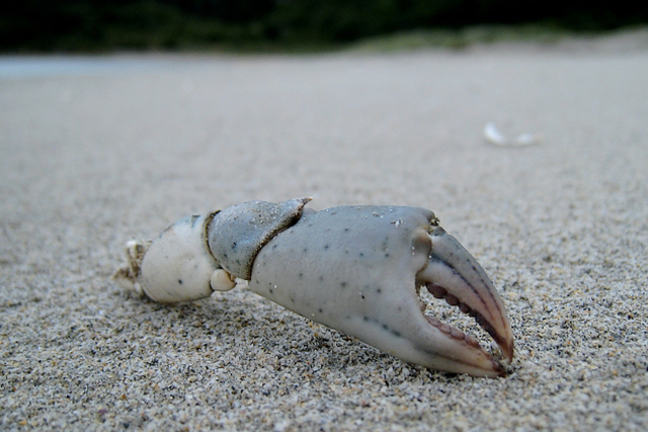4.19

## UNSW SydneyA crabless claw

# Allometry and the Fiddler Crab's Claw

The study of how things scale in biology, usually with respect to body size, is called allometry. The term was introduced by Huxley & Tessier in 1936 in their study of the relationship between the size of a Fiddler crab and its rather extraordinarily large claw.

In this step we have another look at how log-log plots are used to detect and describe power law relations in biology.

## The Fiddler crab

With a log-log scale, we employ a logarithmic scale for both the $\normalsize{x}$ and $\normalsize{y}$ axes. Here is an application to try and understand the curious case of the Fiddler crab:Fiddler crab By The Photographer (Own work) [CC0], via Wikimedia Commons

Fiddler crabs are small crabs that live in the sand. The males have a rather large claw. The bigger the crab, the bigger the claw. Let’s use a table of values of claw size versus crab size.

Crab Size (mm) Claw Size (mm)
3.70 2.12
4.47 2.85
5.08 3.48
6.07 4.57
7.03 5.72
7.80 6.91
8.38 7.64
9.35 9.05
10.24 10.46
11.13 11.83
11.81 13.01
12.41 14.01
13.71 16.61
14.13 17.48
15.31 19.39
16.05 21.08

Here is the corresponding plot of this data:It is not so clear what the relationship between these two variables is from this graph. If we instead plot $\ln\left(\mbox{Crab}\right)$, and $\ln\left(\mbox{Claw}\right)$ the relationship looks different.We see quite clearly that there is a linear relationship here, so that for some constants $\normalsize m$ and $\normalsize b$ we have

The slope $\normalsize m$ of the line is called the allometric constant. Biologists Huxley and Tessier measured this to be $\normalsize{1.57}$. Now, if we exponentiate this equation we get a power law for the relationship between Crab size and Claw size:

where $\normalsize A = e^b$ is some constant. The fact that the exponent here is bigger than one is telling us that the Claw size is growing proportionally faster than the Crab size. This seems to be quite surprising.

Q1 (E): Use the table of Crab sizes and Claw sizes to estimate a value for $\normalsize A$.

## Discussion

Do you know of any other good examples of power laws in unexpected settings in biology?

from which we deduce that $\normalsize A \approx 0.27$.